# Chebyshev iteration method

(diff) ← Older revision | Latest revision (diff) | Newer revision → (diff)

An iterative algorithm for finding a solution to a linear equation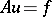(1)

that takes account of information about the inclusion of— the spectrum of the operator— in a certain set, and uses the properties and parameters of those polynomials that deviate least from zero onand are equal to 1 at 0.

The most well-developed Chebyshev iteration method is obtained when in (1),is a linear self-adjoint operator and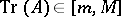, where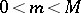are the boundary points of the spectrum; then the Chebyshev iteration method uses the properties of the Chebyshev polynomials of the first kind,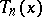. For this case one considers two types of Chebyshev iteration methods: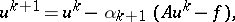(2)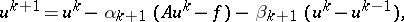(3)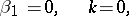in which for a givenone obtains a sequenceas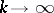. In (2) and (3)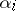and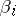are the numerical parameters of the method. If, then the initial errorand the error at the-th iteration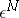are related by the formula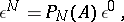where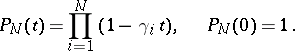(4)

The polynomialsare calculated using the parameters of each of the methods (2), (3): for method (2)(5)

where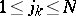are the elements of the permutation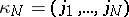, while for method (3) they are calculated from the recurrence relations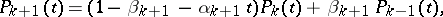(6)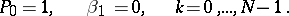HereThe methods (2) and (3) can be optimized on the class of problems for whichby choosing the parameters such that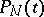in (4) is the polynomial least deviating from zero on. It was proved in 1881 by P.L. Chebyshev that this is the polynomial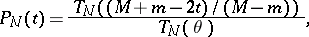(7)

where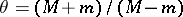. Then(8)

where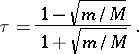Substituting (7) forin (6), the parameters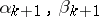of the method (3) are determined: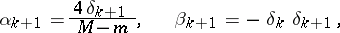(9)

where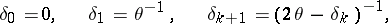(10)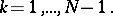Thus, computingand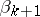by the formulas (9) and (10), one obtains the Chebyshev iteration method (3) for which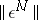is optimally small for each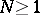.

To optimize (2) for a given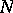, the parameters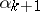are chosen corresponding to the permutation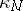in formula (5) in such a way that (7) holds, that is,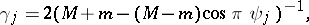(11)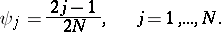Then afteriterations, inequality (8) holds for.

An important problem for smallis the question of the stability of the method (2), (5), (11). An imprudent choice ofmay lead to a catastrophic increase in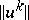for some, to the loss of significant figures, or to an increase in the rounding-off errors allowed on intermediate iteration. There exist algorithms that mix the parameters in (11) and guarantee the stability of the calculations: for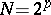see Iteration algorithm; and forone of the algorithms for constructingis as follows. Let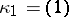, and suppose that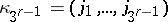has been constructed, then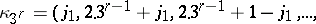(12)There exists a class of methods (2) — the stable infinitely repeated optimal Chebyshev iteration methods — that allows one to repeat the method (2), (5), (11) afteriterations in such a way that it is stable and such that it becomes optimal again for some sequence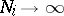. For the case, it is clear from the formula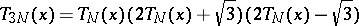(13)

that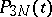agrees with (11). If afteriterations one repeats the iteration (2), (5), (11) further, taking for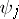in (11) thevalues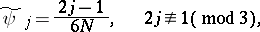(14)

then once again one obtains a Chebyshev iteration method after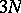iterations. To ensure stability, the set (14) is decomposed into two sets: in the-th set,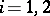, one puts the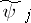for whichis a root of the-th bracket in (13); within each of the subsets theare permuted according to the permutation. For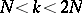one substitutes elements of the first set in (5), (11), and for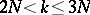one uses the second subset; the permutation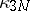is defined in the same way. Continuing in an analogous way the process of forming parameters, one obtains an infinite sequence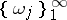, uniformly distributed on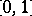, called a-sequence, for which the method (2) becomes optimal with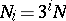and(15)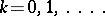The theory of the Chebyshev iteration methods (2), (3) can be extended to partial eigen value problems. Generalizations also exist to a certain class of non-self-adjoint operators, when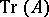lies in a certain interval or within a certain domain of special shape (in particular, an ellipse); when information is known about the distribution of the initial error; or when the Chebyshev iteration method is combined with the method of conjugate gradients.

One of the effective methods of speeding up to the convergence of the iterations (2), (3) is a preliminary transformation of equation (1) to an equivalent equation of the form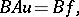and the application of the Chebyshev iteration method to this equation. The operatoris defined by taking account of two facts: 1) the algorithm for computing a quantity of the form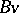should not be laborious; and 2)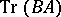should lie in a set that ensures the fast convergence of the Chebyshev iteration method.